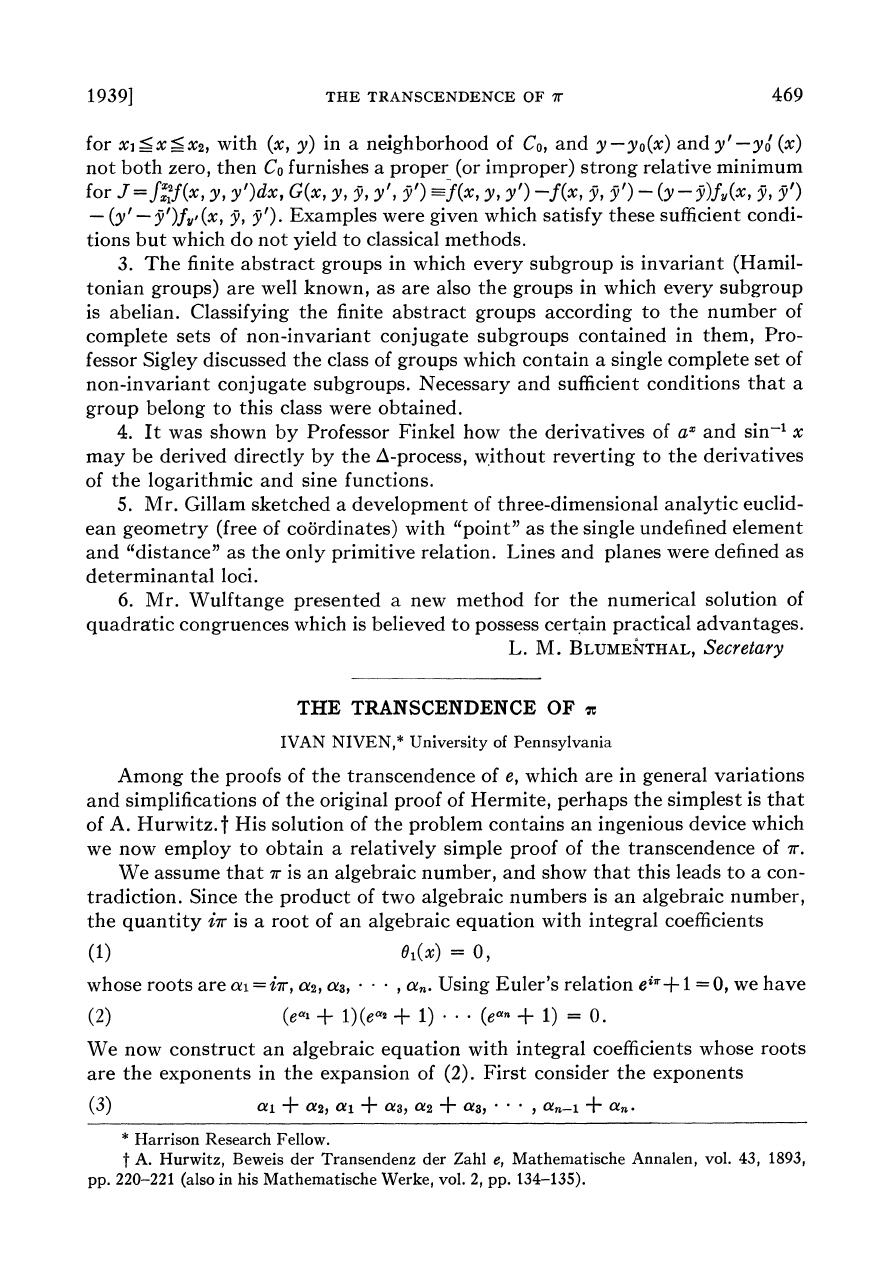A transcendental number is a real or complex number that is not alg...
Ivan Niven was a Canadian-American mathematician, specializing in n...
It's interesting that we know $e$ and $\pi$ are transcendental numb...
It's actually not obvious that the product (or sum) of 2 algebraic ...
Is $i$ an algebraic number?
We showed above that the sum of 2 algebraic numbers is also an alge...
Note that if we expand expression (2) we get $$1+ ...+e^{\alp...$$ \frac{d(e^{-x}F(x))}{dx}=-e^{-x}F(x)+e^{-x}F'(x)=\\ =-e^{-x}F(...
If we make the substitution $\xi = \tau x$ the integration limits ...
How does he end up with $kF(0)$?
This is not difficult to see because of the $x^{p-1}$ factor and al...
Note that $f(x)$ can be written as $$f(x)=\frac{c^sx^{p-1}(cx... Using expression (11)$$ F(\beta_j)=f(\beta_j)+f^{(1)}(\beta_j)...
As shown in a comment above, $f(x)$ can be written as $$f(x)=... Isn't a \tau missing? I mean, shouldn't the integral be$$\int_...
We have finally reached a contradiction since the left side of (12)...1939]
THE TRANSCENDENCE
OF
ir
469
for
XI-
X_X2,
with (x, y)
in a
neighborhood
of
CO,
and
y-yo(x)
and
y'-yoi(x)
not both
zero,
then
CO
furnishes
a proper
(or improper)
strong relative
minimum
for Jf=
f2f(x,
y, y')dx,
G(x, y,
y,
y,
y')-f(x,
y
y, y')-
)-(y-y)fV,(x,
y, y')
-
(y'
-')fy,
(x, y,
y'). Examples
were given
which
satisfy
these
sufficient
condi-
tions but
which do
not
yield
to classical
methods.
3. The finite
abstract
groups
in
which
every
subgroup
is invariant
(Hamil-
tonian
groups) are
well
known, as are also
the groups in which every
subgroup
is abelian. Classifying
the finite abstract
groups
according
to the number
of
complete
sets
of
non-invariant
conjugate
subgroups
contained
in them,
Pro-
fessor Sigley discussed
the class of groups
which
contain a
single complete
set of
non-invariant
conjugate
subgroups.
Necessary
and
sufficient
conditions
that a
group
belong to
this class
were obtained.
4. It
was shown
by
Professor Finkel how the
derivatives
of ax
and
sin-' x
may
be derived
directly by
the A-process,
without
reverting
to the derivatives
of
the
logarithmic
and sine functions.
5.
Mr. Gillam
sketched
a development
of three-dimensional
analytic
euclid-
ean
geometry
(free
of
coordinates)
with
"point"
as the single
undefined
element
and "distance"
as the
only primitive
relation.
Lines
and planes
were defined
as
determinantal
loci.
6. Mr. Wulftange
presented
a new
method
for the numerical
solution of
congruences
which
is
believed
to possess
certain practical
L.
M.
BLUMENTHAL,
Secretary
THE
TRANSCENDENCE
OF
c
IVAN
NIVEN,*
University
of Pennsylvania
Among the
proofs of
the transcendence
of e, which
are in
general variations
and simplifications
of the original
proof
of
Hermite,
perhaps
the
simplest
is
that
of A.
Hurwitz.t
His solution
of the problem
contains
an ingenious
device
which
we
now employ
to obtain
a
relatively
simple proof
of the transcendence
of 7r.
We assume
that
ir
is
an
algebraic
number,
and
show
that
a
con-
the
product
of two
algebraic
numbers
is an
algebraic
number,
the
quantity
i7r
is a
root
of an algebraic
equation
with
integral
coefficients
(1)
01(x)
=
O,
whose
roots
are
aci=ir,
a2, a3,
* *
a.n
Using Euler's
relation e\$r+1
=0,
we
have
(2)
(eal
+
1)(ea2
+
1) *
(ean
+
1)
=
0.
We now
construct an
algebraic
equation
with integral
coefficients whose
roots
are
the
exponents
in the
expansion
of
(2).
First consider
the
exponents
(3)
ai
+
a2,
ai
+
as,
a2
+
C
.
.
*
an-1
+ an
*
Harrison
Research Fellow.
t
A.
Hurwitz,
Beweis der Transendenz
der
Zahl
e,
Mathematische
Annalen,
vol.
43,
1893,
pp. 220-221
(also in his Mathematische
Werke,
vol. 2, pp.
134-135).